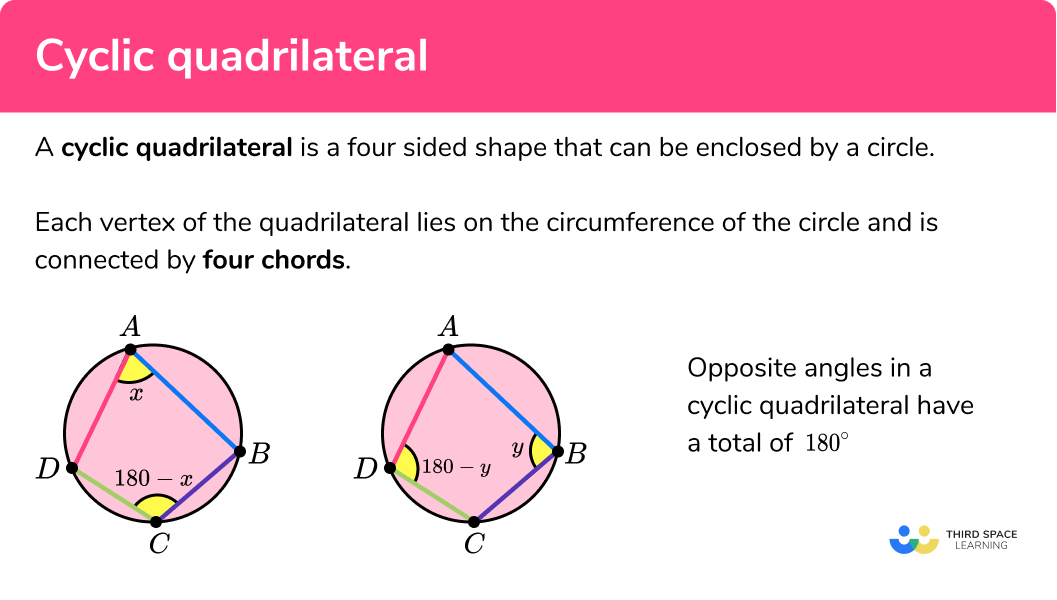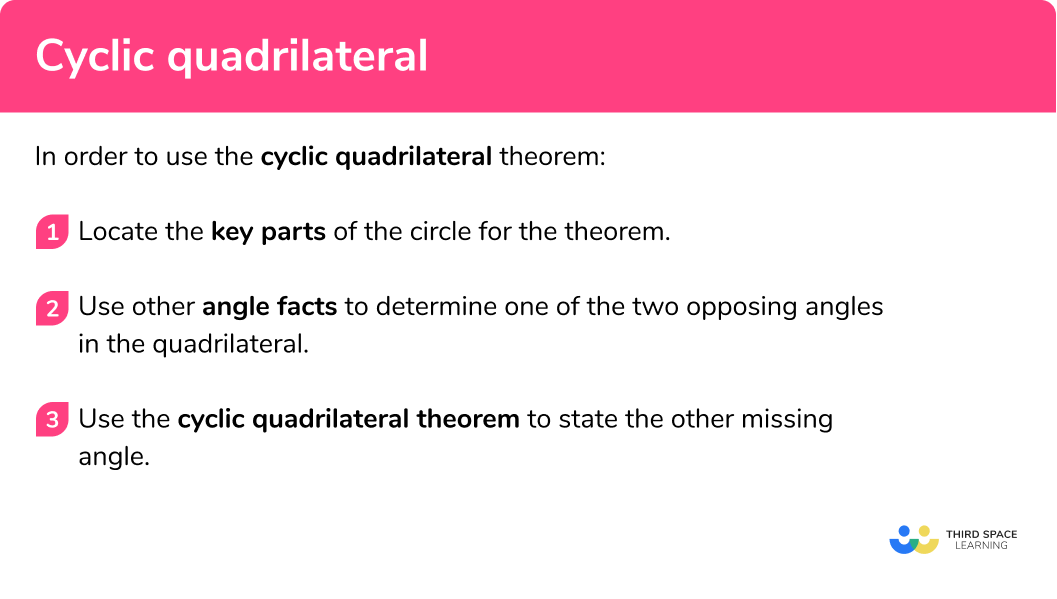Here we will learn about the circle theorem involving cyclic quadrilaterals, including its application, proof, and using it to solve more difficult problems.

There are also circle theorem worksheets based on Edexcel, AQA and OCR exam questions, along with further guidance on where to go next if you’re still stuck.

## What is a cyclic quadrilateral?

A cyclic quadrilateral is a four sided shape that can be inscribed into a circle. Each vertex of the quadrilateral lies on the circumference of the circle and is connected by four chords.

The opposite angles of a cyclic quadrilateral have a total of 180°.

In the diagrams above, the points A, B, C, and D are on the circumference of the circle.

The opposite angles of a cyclic quadrilateral have a total of 180° and so they are supplementary to one another. This means that the angle at A plus the angle at C is equal to 180° , and the angle at B plus the angle at D equal 180° .

This also highlights that angles inside a cyclic quadrilateral total 360° , which is the same property for any quadrilateral.

### What is a cyclic quadrilateral?### Key parts of a circle needed for this theorem

• The centre of the circle is a point that locates the middle of a circle (the midpoint of the circle).
• The circumference of the circle is the distance around the edge of the circle.
• A chord is a straight line that meets the circumference in two places. The longest chord in a circle is the diameter.

### Proving that opposite angles of a cyclic quadrilateral total 180°

To be able to prove that opposite angles of a cyclic quadrilateral total 180° , you need to know the following circle theorems:

• The angle at the centre is twice the angle at the circumference

## How to use the cyclic quadrilateral theorem

In order to use the cyclic quadrilateral theorem:

1. Locate the key parts of the circle for the theorem.
2. Use other angle facts to determine one of the two opposing angles in the quadrilateral.
3. Use the cyclic quadrilateral theorem to state the other missing angle.

### How to use the cyclic quadrilateral theoremTangent of a circle is one of 7 circle theorems you will need to know. You may find it helpful to start with our main circle theorems page and then look in detail at the rest.

### Example 1: standard diagram

ABCD is a cyclic quadrilateral. Calculate the size of angle BCD :

1. Locate the key parts of the circle for the theorem.

Here we have:

• The angle BAD = 51°
• The angle BCD = θ

2Use other angle facts to determine one of the two opposing angles in the quadrilateral.

We already know that BAD = 51° so we do not need to use any other angle fact to determine this angle for this example.

3Use the cyclic quadrilateral theorem to state the other missing angle.

As opposite angles of a cyclic quadrilateral total 180° , we can calculate the size of angle BCD :

$BCD=180-51\\ BCD=129^{\circ}$

### Example 2: unnecessary information

ABCD is a cyclic quadrilateral. Calculate the size of angle BAD :

Locate the key parts of the circle for the theorem.

Use other angle facts to determine one of the two opposing angles in the quadrilateral.

Use the cyclic quadrilateral theorem to state the other missing angle.

### Example 3: angles in a semicircle

ABCD is a cyclic quadrilateral with center O . Calculate the size of angle ABC .

Locate the key parts of the circle for the theorem.

Use other angle facts to determine one of the two opposing angles in the quadrilateral.

Use the cyclic quadrilateral theorem to state the other missing angle.

### Example 4: isosceles triangles

ABCD is a cyclic quadrilateral inside a circle with centre O . BOC is the inscribed angle of the chord BC . Calculate the size of angle ADC .

Locate the key parts of the circle for the theorem.

Use other angle facts to determine one of the two opposing angles in the quadrilateral.

Use the cyclic quadrilateral theorem to state the other missing angle.

### Example 5: angles in triangles

The circle below contains the quadrilateral ABCD . Calculate the size of angle CAD :

Locate the key parts of the circle for the theorem.

Use other angle facts to determine one of the two opposing angles in the quadrilateral.

Use the cyclic quadrilateral theorem to state the other missing angle.

### Example 6: tangents to a circle

ABCD are vertices of the quadrilateral inscribed in a circle with centre E . BF and CF are tangents to the circle with centre E . Calculate the size of angle CDE .

Locate the key parts of the circle for the theorem.

Use other angle facts to determine one of the two opposing angles in the quadrilateral.

Use the cyclic quadrilateral theorem to state the other missing angle.

### Common misconceptions

• Angle at the centre is supplementary to opposing angle

As the shape is a quadrilateral, the angle at the centre is assumed to be supplementary and add to 180° .

• Opposite angles are the same

As angles in the same segment are equal, the opposing angles in a quadrilateral are assumed to be equal. The only case of this is when both angles are 90° . As angles in the same segment are equal, the opposing angles in a quadrilateral are assumed to be equal. The only case of this is when both angles are 90° .

• Opposite angles are double

Similar to the angle at the centre theorem, it is assumed that the opposing angle in a cyclic quadrilateral is double or half of the other.

• Opposite angles don’t add up to 180°

The angles in a cyclic quadrilateral are calculated incorrectly (usually after forming and solving an equation and so the opposite angles do not add to 180° .

1. ABCD is a cyclic quadrilateral. Calculate the size of angle ABC .117^{\circ}63^{\circ}126^{\circ}127^{\circ}180-63=117^{\circ} (opposite angles of a cyclic quadrilateral total 180^{\circ} )

2. The circle with centre O has four points on the circumference at A, B, C, and D . Without using a protractor, calculate the size of angle BCD marked \theta .85^{\circ}95^{\circ}170^{\circ}94^{\circ}180-85=95^{\circ} (opposite angles of a cyclic quadrilateral total 180^{\circ} )

3. ABCD is a cyclic quadrilateral. The line AC passes through the centre O and angle BAD = 107^{\circ} . Calculate the size of angle \theta .46^{\circ}73^{\circ}61^{\circ}12^{\circ}• 180-107=73^{\circ} (opposite angles of a cyclic quadrilateral total 180^{\circ} )
• 73-61=12^{\circ}

4. The points A, B, and D are on the circumference of a circle with centre C . Calculate the size of the interior angle BAD .122^{\circ}148^{\circ}61^{\circ}119^{\circ}• 360-122=238^{\circ} (angles around a point total 360^{\circ} )
• 238\div2=119^{\circ}

5. ABCD is a cyclic quadrilateral. The chords AC and BD intersect at the point E . calculate the size of angle ACD .39^{\circ}57^{\circ}92^{\circ}123^{\circ}• 180-(18+39)=123^{\circ} (opposite angles of a cyclic quadrilateral total 180^{\circ} )
• ACB = 31^{\circ} as angles in the same segment are equal.
• 123-31=92^{\circ}

6. The circle with centre O contains a cyclic quadrilateral. The line EF is a tangent at point D where angle CDF = 43^{\circ} . Calculate the size of angle OBC .43^{\circ}55^{\circ}78^{\circ}36^{\circ}• ODF = 90^{\circ} so OCD = 90-43 = 47^{\circ} (tangent and radius meet at 90^{\circ} )
• BOD=78\times2=156^{\circ} (angle at the centre is twice the angle at the circumference)
• BCD=180-78=102^{\circ} (opposite angles of a cyclic quadrilateral total 180^{\circ} )
• OBC=360-(47+156+102)=55^{\circ} (angles in a quadrilateral total 360^{\circ} )

1. PQRS is a quadrilateral inscribed in a circle. Calculate the value of x .(3 marks)

180 – 124 = 56^{\circ}

(1)

4x=56^{\circ}

(1)

x=14^{\circ}

(1)

2. Below is a semicircle:(a) Malik says “the angle = 90^{\circ} because the angle in a semicircle is equal to 90 degrees”. Explain why Malik is not correct.

(3 marks)

(a)

The angle in a semicircle is equal to 90^{\circ} when the angle is subtended from the chord A and B .

The angle BCD is subtended from the chord BD and so it does not equal 90^{\circ} as BD is not the diameter of the semicircle.

(1)

(b)

180 – 52 = 128^{\circ}

(1)

Opposite angles in a cyclic quadrilateral total 180^{\circ}

(1)

3. (a) ABCD is an inscribed quadrilateral within a circle. AC and BD intersect at the point E . Use the information given to calculate x , the angle DCE .(9 marks)

(a)

(1)

Angles in the same segment are equal

(1)

CDE = 87 – 7 = 80^{\circ}

(1)

CED = 44^{\circ}

(1)

Vertically opposite angles are equal

(1)

DCE = 180 – (80+44) = 56^{\circ}

(1)

Angles in a triangle total 180^{\circ}

(1)

(b)

180 – (56+7) = 117^{\circ}

(1)

Opposite angles in a cyclic quadrilateral total 180^{\circ}

(1)

## Learning checklist

You have now learned how to:

• Apply and prove the standard circle theorems concerning angles, radii, tangents and chords, and use them to prove related results

## Still stuck?

Prepare your KS4 students for maths GCSEs success with Third Space Learning. Weekly online one to one GCSE maths revision lessons delivered by expert maths tutors.

Find out more about our GCSE maths tuition programme.